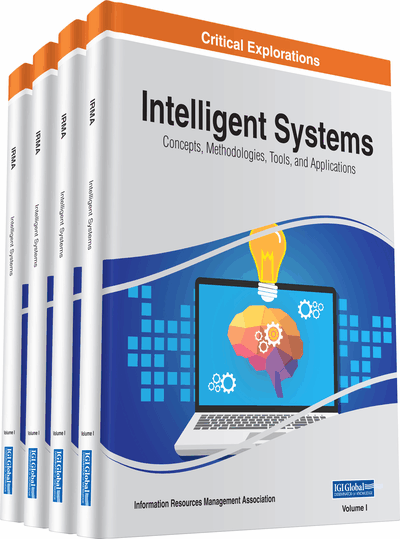# Towards a New Model for Causal Reasoning in Expert Systems

M. Keith Wright (University of Houston Downtown, USA)
DOI: 10.4018/978-1-5225-5643-5.ch005
Available
\$29.50
No Current Special Offers

## Abstract

This paper presents ideas for improved conditional probability assessment and improved expert systems consultations. It cautions that knowledge engineers may sometimes be imprecise when capturing causal information from experts: their elicitation questions may not distinguish between causal and correlational expertise. This paper shows why and how such models cannot support normative inferencing over conditional probabilities as if they were all based on frequencies in the long run. In some cases, these probabilities are instead causal theory-based judgments, and therefore are not traditional conditional probabilities. This paper argues that these should be processed as if they were causal strength probabilities or causal propensity probabilities. This paper reviews the literature on causal and probability judgment, and then presents a probabilistic inferencing model that integrates theory-based causal probabilities with frequency-based conditional probabilities. The paper also proposes guidelines for elicitation questions that knowledge engineers may use to avoid conflating causal theory-based judgment with frequency based judgment.
Chapter Preview
Top

## Introduction

Probabilistic causal knowledge plays a major role in many types of decision support systems including expert systems (Chan et al. 2011; Duda, Hart, & Nelson, 1976) influence diagrams (Howard & Matheson, 1984), bayesian networks (Pearl, 1988); and clinical decision-making (Luca et al., 2011; Rodríguez-González, et al., 2011; Speigelhalter (1987); Shortliffe & Buchanan, 1975).

For use in expert systems, causal relations are often captured in the form of a series of conditional probabilities. Under a frequency interpretation of probability, the probabilities can be interpreted unambiguously and illustrated via Venn diagrams. However, probability judgments are not always based on frequency data. Instead, they are sometimes suppositions about single event propositions. These one-of-a-kind subjective judgments have been termed causal theory-based (Jennings, Amabile & Ross, 1952; Ajzen, 1977). An example of a typical assessment question requiring causal theory-based judgment is, “What is the probability of U.S. gasoline prices exceeding \$25.00 per gallon given there is widespread war in the Middle East?”

Although much has been written on the problem of assessing frequency based unconditional probabilities (e.g., See Moskowitz & Wallenius, 1984; Hogarth, 1975; Spetzler & Merkhoffer, 1987).), relatively little attention has been focused on the assessment of causal theory-based probabilities. The following results of mathematical probability give the knowledge engineer several normative choices when assessing the causal relationship between two events “c” (cause) and “e” (effect):

• 1.

p(c ∩ e) = p(c | e) * p(e) = p(e | c) * p(c);

• 2.

p(c | e) = p(c & e) / p(e);

• 3.

p(e) = ∑ p (e & ci) where ci is a member of the exhaustive set of possible mutually exclusive causes of effect (e).

Conventional wisdom holds that the assessment of conditional probability is easier for the subject than the assessment of the corresponding unconditional probabilities or joint probabilities. (e.g. See the discussions of probability judgment in Ravinder et al. (1988)). However, Moskowitz & Wallenius (1984) points out that:

The subjective assessment of conditional probability, P (x | y) is intricate and subtle posing additional problems not encountered in unconditional probability assessment. These normative violations are not simply random or minor errors that are expected in any subjective elicitation, but are errors of considerable magnitude resulting from systematic perceptual and cognitive biases. Moreover, even statistically mature experts are highly susceptible to these errors, which have been ignored in previous studies on conditional and joint probability assessments. (Wallenius, 1984)

Dyer (1980) asked a group of oil company experts for estimates of the following probabilities:

• P (e) = the probability that world oil prices will be higher in 1990;

• P(c) = the probability that environmental regulation will be stricter in 1990;

• P (e | c) = the probability that oil prices will be higher in 1990 given that environmental regulations are stricter in 1990.

## Complete Chapter List

Search this Book:
Reset